Sign inRelationships Parenting Wedding

Parenting

Upbringing

# Dear Parents, See 9 Maths Tricks No Teacher Would Teach Your Kids In School.The truth is that a lot of students don't love mathematics because of its complexities. But like every other subject that are taught in school, mathematics has its own hidden tricks. I don't usually blame anyone that has issues with solving mathematics because there are some mathematics tricks that will really facilitate your work, but sadly no mathematics teacher would teach you this tricks in school.However, this informative article contains nine(9) mathematics tricks that will really help your kids works smarter, easier, and faster. Read Carefully And Learn.

1. How to find the square of two-digits number that ends with 5.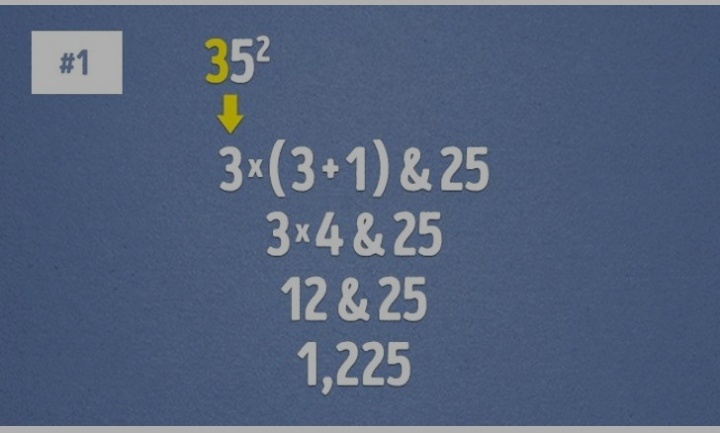As shown above, all you need to do is multiply the first number by itself plus one(1). After that, write 25. Easy right?

2. How to multiply by 4.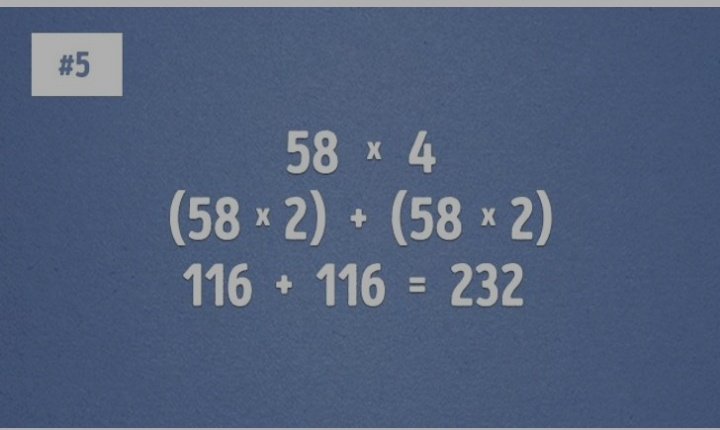For simplicity purpose, multiply the number by 2 (in two places), and then add both solutions together.

3. How to easily find the cube root of a large number.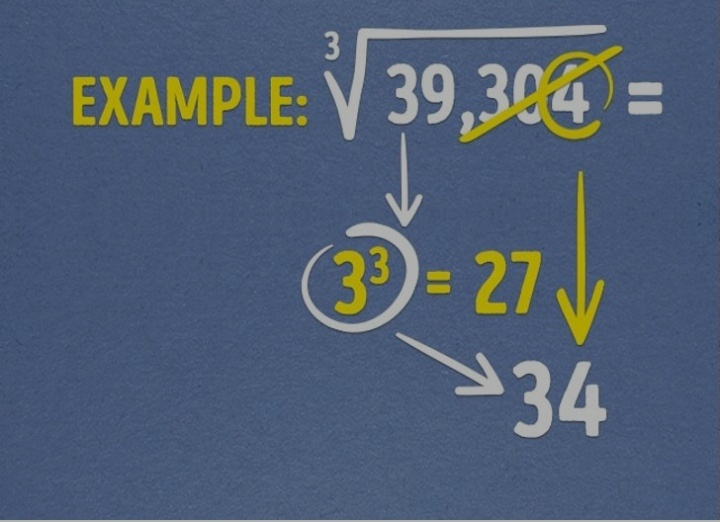First of all, know that you only have to know the the cubes of numbers between 1 to 10.

Now, note the last digit of the number because it will will be the last digit of your answer (4 is the last digit in the example).

After that, snub the last three digits (304 in the example).

Now, check what remains(39 remains in the example), then find which of the first 10 cubes is closest to the remaining digits without going over it( note that 39 is the remaining digits in the example).

From the example, 3 is the perfect number because 3³ is 27 ( 27 is the closest to 39).

In the example the answer is 34.

4. How to easily deduct numbers from 1000.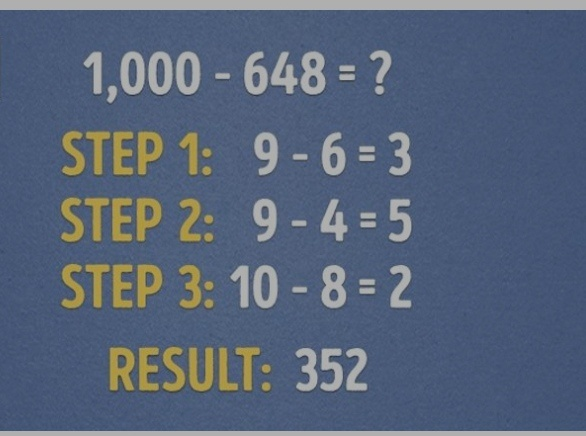This is quite easy, all you have to do is deduct all digits of the number from 9, and then the last digit should be subtracted from 10. Easy right?

5. How to multiply large numbers.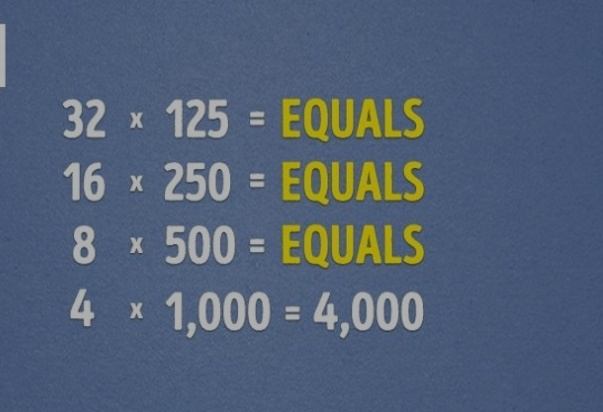The trick involved is simply regrouping the numbers for simplicity purpose. For instance, If you're told to multiply 32 by 125, simply regroup the numbers as shown in the picture above.

6. See how you can do a multiplication by 9 easily.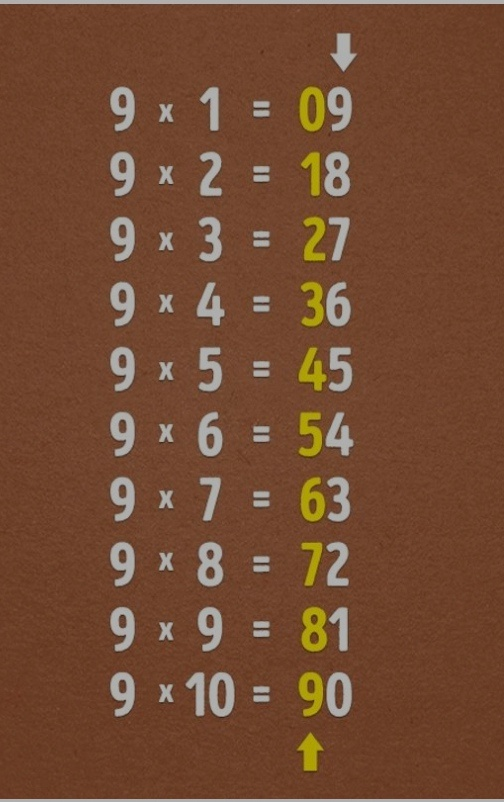Take note of the ascending and descending numbers.

7. How to calculate the percentage of a number.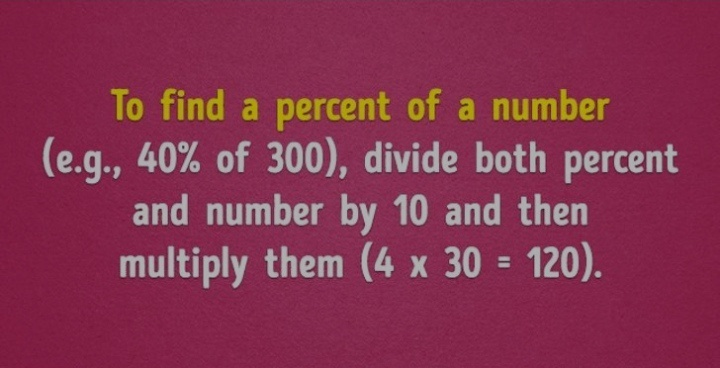This is easy. To calculate the percentage of any number, simply divide both the percent and the number itself by 10. After that multiply them together. An example is shown below.

8. An fast and simple way to carry out multiplication by 11 is shown below (32 X 11).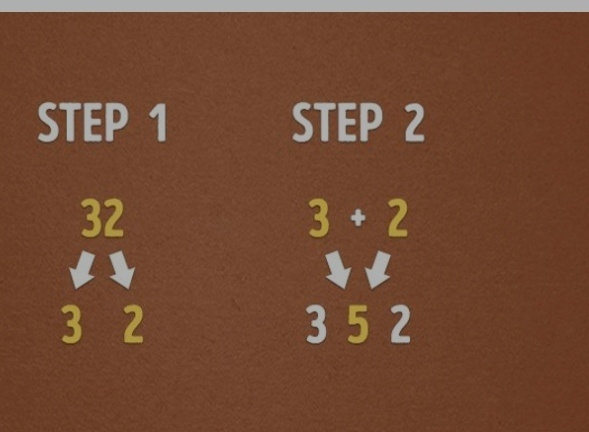9. How to divide by 5 easily.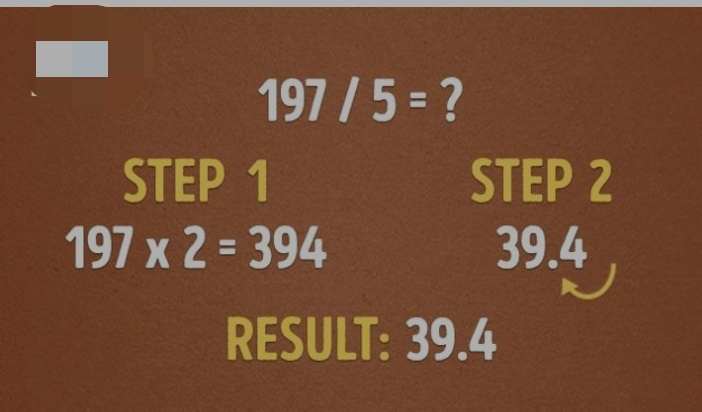I am happy to inform you that this is one of the easiest problems you can ever solve in mathematics.

All that you have to do is multiply the number by two (2). After that, divide the result by 10 i.e shift the decimal point.

An example is shown below.